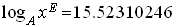Mathman 24 - Wiscompton Loggers by Karlee Patzlsberger, Allie Deming, and Nathan KempfTerrain: Grassy park Difficulty: Depends on math ability Placed by: The Dragon (Mathman) and his Honors Advanced Algebra students of 2005-06 Location: Kinderberg Park, Germantown, WI County: Washington, WI Materials needed: Calculator, Compass, Writing Utensil, Personal Stamp, Inking PensStudents and parents of students should read my introduction to letterboxing before seeking the boxes.

These clues rely on the use of matrices to encode/decode clues.  Go to this page to learn how to do this on a free graphics calculator simulator or to do it by hand.

Be sure to read through all the clues before looking for the boxes so you know what is expected of you.

Clues:

Start in front of the park sign on the corner of Preserve Parkway and Buckthorn Dr.

1.   On Pascal’s Triangle, find the diagonal that includes the time the park opens and the chapter number for the rules of the park. The next term on that diagonal will be how many steps you should travel down the path into the park: _____

2.   Look to your right and count the number of soccer goals, the number of small trees there are along the road (Preserve Parkway) and the number softball field(s) (to the left).

Soccer goals: A = _____               Small pines: B = _____          Baseball fields: C = _____

**Note: There may be some confusion to the answer for B.  Just to be sure, it should be one more than the number of letters in the name of this location.

Enter the numbers into the quadratic equation Ax2 + Bx + C = 0 and find the value of the discriminant: _____ Take that many more steps down the path.

3.   You’re doing well! Reward yourself by taking a rest on the yellow bench. While resting, solve the following for the positive answer to find how many steps to walk counter-clockwise around the woodchips:

x2 + 58x - 120 = 0

While you are on your way around, collect the following information:

 D=_____ The number of "rockers" (Hint: one is a motorcycle) E=_____ The number of "diggers". F=_____ The number of park buildings in the area. G=_____ The number of fire hydrants in the area. H=_____ The number of black swings. I=_____ The number of red swings. J=_____ The number of red slides. K=____ The number of different colored poles in the waterworks. L=_____ The number of yellow benches in the immediate area of the waterworks.

4.  Once you’ve completed this task, rest at the yellow bench directly SE of the red leafed tree (red maple). While resting take the square root of the closing time of the water park, and multiply it by x, after solving log4x=1.5.

Take that answer and walk that many steps toward the Germantown water tower.  Then do it again! Walk to the nearest light pole.  Find the number on the base of that pole: M = _____  and the number of letters in the company that manufactured the light poles: N = ______

5. Return to the bench by the red maple to use all the numbers that you collected to finish the math you need to do.

To find P = ______, solve for x:To find Q = ______, add F to the sum of the first eight terms of the arithmetic sequence which has M as its first term and H as its common difference.

To find R = _______, find t45 of the arithmetic sequence which has J and the first term and (K-G) as the common difference.

To find S = _______, find the value of the determinant:To find the "object", subtract the following matrices and then decode it using the key:KEY:
SPACE=0  A=1  B=2  C=3  D=4  E=5  F=6  G=7  H=8  I=9  J=10  K=11  L=12  M=13  N=14  O=15  P=16  Q=17  R=18  S=19  T=20  U=21  V=22  W=23  X=24  Y=25  Z=26

BEFORE RETRIEVING THE BOX, BE SURE NO ONE IS AROUND TO SEE YOU TAKE THE BOX OUT!  DO NOT BRING ATTENTION TO YOURSELF OR THE HIDING LOCATION OF THE BOX!

Now that you're all rested up and have the numbers you need, take a hike to the top of the hill behind you.  Once at the top, position yourself so that one of the entrances to the park from Preserve Parkway is at a bearing of P degrees and the other is at a bearing of Q degrees (along a line dividing the hill in half north to south).  Face the "object" and take R steps in that direction.  You should come to a "bridge." Take S steps from the start of the "bridge".  The box is under the large Ohio shaped rock to your right.  Just move the smaller loose stones on its left side to get to the box.  After stamping up, replace the box and the loose stones so the box can’t be seen, but make it look natural.

Good luck!

Before you set out read the waiver of responsibility and disclaimer.

LbNA Home